# 吴恩达机器学习1

## 什么是机器学习

$A\ computer\ program\ is\ said\ to\ learn\ from\ experience\ E\ with$

$respect\ to\ some\ class\ of\ tasks\ T\ and\ performance\ measure\ P,\ if\$

$its\ performance\ at\ tasks\ in\ T,\ as\ measured\ by\ P,\ improves\ with\$

$experience\ E.$

$E:$ 下过很多跳棋的经验

$T：$下跳棋的任务

$P ：$下一局赢下跳棋的可能性

### 什么是监督学习

$In\ supervised\ learning,\ we\ are\ given\ a data\ set\ and\ already\ know\$

$what\ our\ correct\ output\ should\ look\ like,having\ the\ idea\ that\ there\$

$is\ a\ relationship\ between\ the\ input\ and\ the\ output.$

$Supervised\ learning\ problems\ are\ categorized\ into\ “regression”\ and\$

$”classification”\ problems.\ In\ a\ regression\ problem,\ we\ are\ trying\ to\$

$predict\ results\ within\ a\ continuous\ output,\ meaning\ that \ we\ are\$

$trying\ to\ map\ input\ variables\ to\ some\ continuous\ function.\ In\ a\$

$classification\ problem,\ we\ are\ instead\ trying\ to\ predict\ results\$

$in\ a\ discrete\ output.\ In\ other\ words,\ we\ are\ trying\ to\ map\ input\$

$variables\ into\ discrete\ categories.\$

### 什么是无监督学习

$Unsupervised\ learning\ allows\ us\ to\ approach\ problems\ with\ little\ or\$

$no\ idea\ what\ our\ results\ should\ look\ like.\ We\ can\ derive\$

$structure\ from\ data\ where\ we\ don’t\ necessarily\ know\ the\ effect\ of\$

$the\ variables.\$

$We\ can\ derive\ this\ structure\ by\ clustering\ the\ data\ based\ on\$

$relationships\ among\ the\ variables\ in\ the\ data.$

$With\ unsupervised\ learning\ there\ is\ no\ feedback\ based\ on\ the\ prediction\ results.$

## 建立符号规则

$m$ 表示训练集数量，对于训练集中的每一项，我们使用$x^{(i)}$表示训练集中的第$i$行输入的变量，使用$y^{(i)}$表示训练集中第$i$行输出的变量 。

## 监督学习

$To\ describe\ the\ supervised\ learning\ problem\ slightly\ more\ formally,\$

$our\ goal\ is,\ given\ a\ training\ set,\ to\ learn\ a\ function\ h\ :\ X\ →\ Y\$

$so\ that\ h(x)\ is\ a\ “good”\ predictor\ for\ the\ corresponding\ value\ of\ y.\$

$For\ historical\ reasons,\ this\ function\ h\ is\ called\ a\ hypothesis.\$

$Seen\ pictorially,\ the\ process\ is\ therefore\ like\ this:\$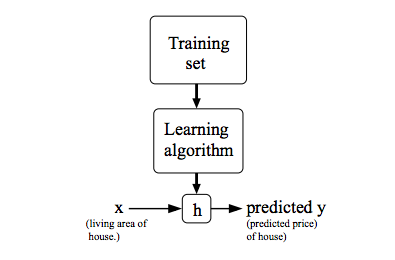### 代价函数

$Goal:\ \min\limits_{\theta_0,\theta_1}J(\theta_0, \theta_1)$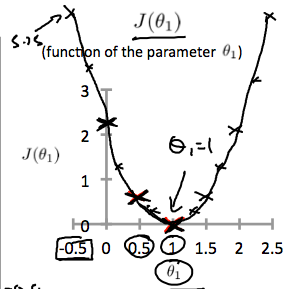### 梯度下降

$The\ way\ we\ do\ this\ is\ by\ taking\ the\ derivative\ (the\ tangential$

$line\ to\ a\ function)\ of\ our\ cost\ function.\ The\ slope\ of\ the\ tangent$

$is\ the\ derivative\ at\ that\ point\ and\ it\ will\ give\ us\ a\ direction$

$to\ move\ towards.\ We\ make\ steps\ down\ the\ cost\ function\ in\ the$

$direction\ with\ the\ steepest\ descent.$

$The\ size\ of\ each\ step\ is\ determined\ by\ the\ parameter\ α,\ which\ is$

$called\ the\ learning\ rate.$

$repeat\ until\ convergence\ \{ \\ \theta_j\ :=\ \theta_j\ - \alpha \ \frac{\partial}{\partial\theta_j}J(\theta_0,\theta_1) (for j=0 and j=1) \\ \}$

$\theta_0$和$\theta_1$同时更新，而不是先更新$\theta_0$，然后再更新$\theta_1$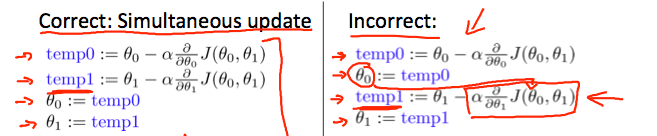$f(x) = \frac{f(x_0)}{0!}+\frac{f’(x_0)}{1!}(x-x_0)+…+\frac{f^{(n)}(x_0)}{n!} + R_n(x)$

$f(x+\Delta x)\approx f(x) + a^t\Delta x$

$\Delta x$是一个向量，$a$也是一个向量,$a^t$就是当前的梯度

$a^t\Delta x= \ge -||a|| \ ||b|| \ge -||a||\epsilon$

#### 梯度下降如何达到最优点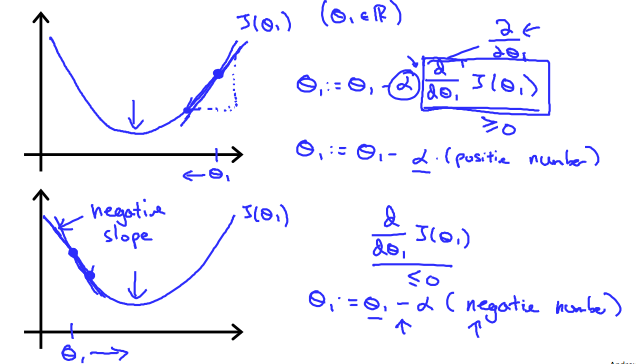$\theta_1 :=\theta_1-\alpha \frac{\partial}{\partial\theta_1}J(\theta_0)$

#### 步距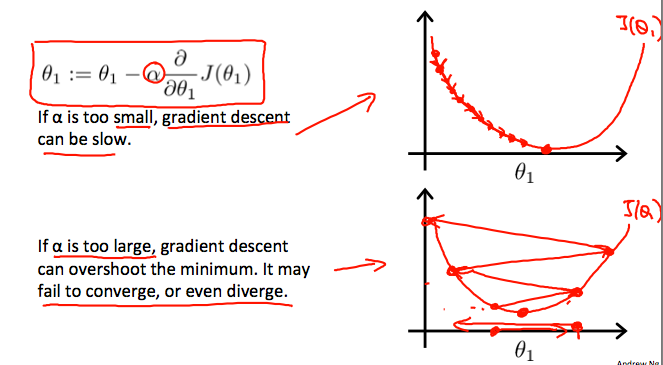#### 梯度下降如何与固定步长相结合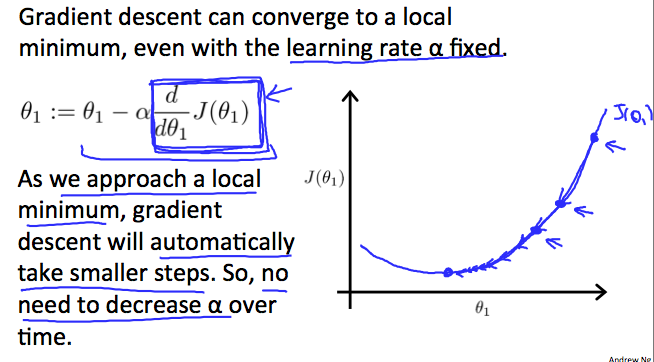#### 梯度下降与代价函数结合

$m$是训练集的大小，$\theta_0$是常量它会随着$\theta_1$同步变化

### 矩阵和向量

$y=\begin{bmatrix} a\\ b\\c\\d\\ \end{bmatrix}$

$y_i$表示第$i$个元素

$y=\begin{bmatrix} y_1\\ y_2\\ y_3\\ y_4\\ \end{bmatrix} , y=\begin{bmatrix} y_0\\ y_1\\ y_2\\ y_3\\ \end{bmatrix}$

#### 矩阵加法

$\begin{bmatrix}1 && 0\\2 && 5 \\ 3 && 1\end{bmatrix} + \begin{bmatrix}4 && 0.5\\2 && 5 \\ 0 && 1\end{bmatrix}=\begin{bmatrix}5 && 0.5\\4 && 10 \\ 3 && 2\end{bmatrix}$

#### 矩阵乘除

$k\times \begin{bmatrix}1 && 0\\2 && 5 \\ 3 && 1\end{bmatrix} = \begin{bmatrix}1k && 0k\\2k && 5k \\ 3k && 1k\end{bmatrix}$

$\begin{bmatrix} A_{11} && A_{12} && A_{13} \\ A_{21} && A_{22} && A_{23}\end{bmatrix} \times \begin{bmatrix} B_{11} && B_{12} \\ B_{21} && B_{22} \\ B_{31} && B_{32} \end{bmatrix}$

$\begin{bmatrix} (A_{11}\times B_{11}+A_{12}\times B_{21}+A_{13}\times B_{31}) && (A_{11}\times B_{12}+A_{12}\times B_{22}+A_{13}\times B_{32}) \\ (A_{21}\times B_{11}+A_{22}\times B_{21}+A_{23}\times B_{31}) && (A_{21}\times B_{12}+A_{22}\times B_{22}+A_{23}\times B_{32}) \end{bmatrix}$

#### 元矩阵

$\begin{bmatrix}1 && 0\\0 && 1\end{bmatrix}$或者 $\begin{bmatrix}1 && 0 && 0\\0 && 1 && 0 \\ 0 && 0 && 1\end{bmatrix}$ 或者更大

$A \cdot I=I\cdot A=A$

#### 逆矩阵

$AA^{-1}=A^{-1}A=I$

#### 矩阵的转置

$A=\begin{bmatrix}1 && 2 && 0 \\ 3 && 5 && 9 \end{bmatrix}$

$A^T=\begin{bmatrix}1 && 3 \\ 2 && 5 \\ 0 && 9 \end{bmatrix}$

#### 矩阵与梯度下降结合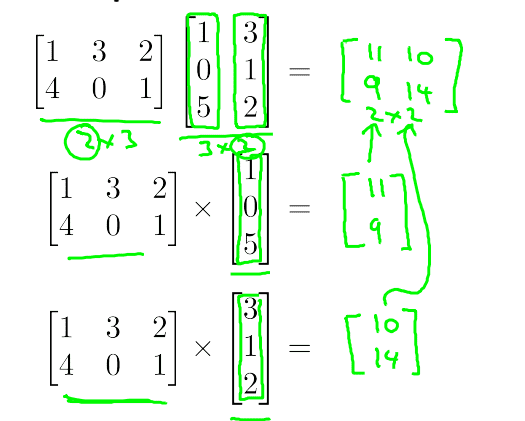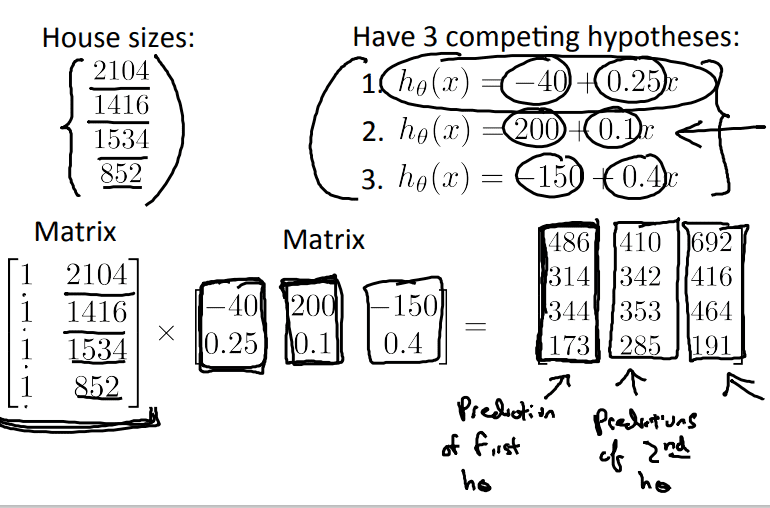目录